# Blog

## 5000+ Members

### MEETUPS

LEARN, CONNECT, SHARE

### JOB POSTINGS

INDEED POSTINGS

Browse through the latest deep learning, ai, machine learning postings from Indeed for the GTA.

### CONTACT

CONNECT WITH US

Are you looking to sponsor space, be a speaker, or volunteer, feel free to give us a shout.

# Automating financial decision making with deep reinforcement learning

Machine learning (ML) is routinely used in every sector to make predictions. But beyond simple predictions, making decisions is more complicated because non-optimal short-term decisions are sometimes preferred or even necessary to enable long-term, strategic goals. Optimizing policies to make sequential decisions toward a long-term objective can be learned using a family of ML models called Reinforcement Learning (RL).

Amazon SageMaker is a modular, fully managed service with which developers and data scientists can build, train, and deploy ML models at any scale. In addition to building supervised and unsupervised ML models, you can also build RL models in Amazon SageMaker. Amazon SageMaker RL builds on top of Amazon SageMaker, adding pre-packaged RL toolkits and making it easy to integrate custom simulation environments. Training and prediction infrastructure is fully managed so you can focus on your RL problem and not on managing servers.

In this post I will show you how to train an agent with RL that can make smart decisions at each time step in a simple bidding environment. The RL agent will choose whether to buy or sell an asset at a given price to achieve maximum long-term profit. I will first introduce the mathematical concepts behind deep reinforcement learning and describe a simple custom implementation of a trading agent in Amazon SageMaker RL. I will also present some benchmark results between two different types of implementation. The first, most straightforward approach consists of an agent looking back at a 10-day window to predict the best decision to make between buying, selling, or doing nothing. In the second approach, I design a time series forecasting deep learning recurrent neural network (RNN) and the agent acts based on forecasts produced by the RNN. The RNN encoder plays the role of an advisor as the agent makes decisions and learns optimal policies to maximize long-term profit.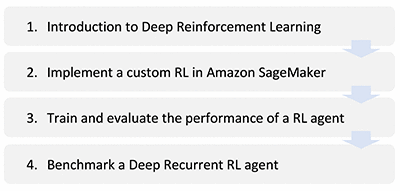The data for this post is an arbitrary bidding system made of financial time series in dollars that represent the prices of an arbitrary asset. You can think of this data as the price of an EC2 Spot Instance or the market value of a publicly traded stock.

## Introduction to deep reinforcement learning

Predicting events is straightforward, but making decisions is more complicated. The framework of reinforcement learning defines a system that learns to act and make decisions to reach a specified long-term objective. This section describes the key motivations, concepts, and equations behind deep reinforcement learning. If you are familiar with RL concepts, you can skip this section.

### Notations and terminology

Supervised learning relies on labels of target outcomes for training models to predict these outcomes on unseen data. Unsupervised learning does not need labels; it learns inherent patterns on training data to group unseen data according to these learned patterns. But there are cases in which labeled data is only partially available, cases in which you can only characterize final targeted outcomes and you’d like to learn how to reach these outcomes, or even cases in which tradeoffs and compromises need be learned given an overall objective (for example, how to balance work and personal life every day to remain happy in the long term). Those are cases where RL can help. Motivations behind RL are indeed cases in which you cannot define a complete supervision and can only define feedback signals based on actions taken, and cases in which you’d like to learn the optimal decisions to make as time progresses.

To define an RL framework, you need to define a goal for a learning system (the agent) that makes decisions in an environment. Then you need to translate this goal into a mathematical formula called a reward function, aimed at rewarding or penalizing the agent when it takes an action and acting as a feedback loop to help the agent reach the predefined goal. There are three key RL components: state, action, and reward; at each time step, the agent receives a representation of the environment’s state st, takes an action at based on st, and receives a numerical reward rt+1based on st+1 = (st, at).

The following diagram presents key components of Reinforcement Learning.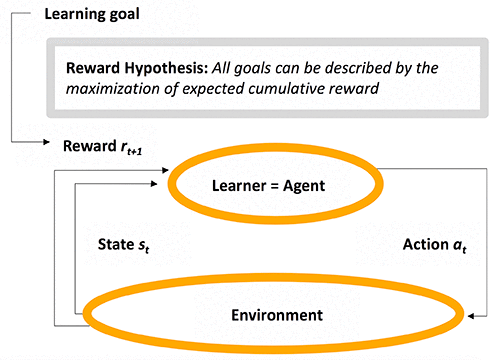Defining a reward function can be easy or, in contrast, highly empirical. For example, in the AWS DeepRacer League, participants are asked to invent their own RL reward function to compete in virtual and in-person races worldwide, because as of this writing, no one knows how to define the best policy for a car to win a race. In contrast, for a trading agent, the reward function can be simply defined as the overall profit accumulated, because this is always a key performance that traders are driving toward.

In most cases, situations in which an agent is confronted may change over time depending on which actions it takes, and thus the RL problem requires to map situations to actions that are best in these particular situations. The general RL problem consists of learning entire sequences of actions, called policies, and noted 𝜋(a|s), to maximize reward.

Before looking at methods to find optimal policies, you can formalize the objective of learning when the goal is to maximize the cumulative reward after a time step t by defining the concept of cumulative return Gt: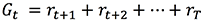When there is a notion of a final step, each subsequence of actions is called an episode, and the MDP is said to have a finite horizon. If the agent-environment interaction goes on forever, the MDP is said to have an infinite horizon; in which case you can introduce a concept of discounting to define a mathematically unified notation for cumulative return: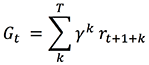If γ = 1 and T is finite, this notation is the finite horizon formula for Gt, while if γ < 1, episodes naturally emerge even if T is infinite because terms with a large value of k become exponentially insignificant compared to terms with a smaller value of k. Thus the sum converges for infinite horizon MDP, and this notation applies for both finite and infinite horizon MDP.

### Optimizing sequential decision-making with RL

There exists a large number of optimization methods that have been developed to solve the general RL problem. They are generally grouped into either policy-based methods or value-based methods, or combination thereof. We’ll focus mostly on the value-based methods as explained below.

#### Policy-based RL

In a policy-based RL, the policy is defined as a parametric function of some parameters θ: 𝜋θ = 𝜋(a|s,θ) and estimated directly by standard gradient methods. This is done by defining a performance measure, typically the expected value of Gt for a given policy, and applying gradient ascent to find θ that maximizes this performance measure. 𝜋θ can be any parameterized functional form of 𝜋 as long as 𝜋 is differentiable with respect to θ; thus a neural network can be used to estimate it, in which case the parameters θ are the weights of the neural network and the neural network predicts entire policies based on input states.

Direct policy search is relatively simple and also very limited because it attempts to estimate an entire policy directly from a set of previously experienced policies. Combining policy-based RL with value-based RL to refine the estimate of Gt almost always improves accuracy and convergence of RL, so this post focuses exclusively on value-based RL.

#### Value-based RL

An RL process is a Markov Decision Process (MDP), which is a mathematical formalization of sequential decision-making for which you can make precise statements. An MDP consists in a sequence (s0, a0, r1, s1, a1, r2, …, sn), or more generally (st, at, rt+1, st+1)n, and the dynamics of an MDP as illustrated in the previous diagram is fully characterized by a transition probability T(s,a,s’) = p(s’|s,a) that defines the probability for any state s to going to any other state s’, and a reward function r(s,a) = 𝔼(r|s,a) that defines the expected reward for any given state s.

Given the goal of RL is to learn the best policies to maximize Gt, this post first describes how to evaluate a policy in an MDP, and then how to go about finding an optimal policy.

To evaluate a policy, define a measure for any state s called a state value function V(s) that estimates how good it is to be in s for a particular way of acting, that is, for a particular policy 𝜋: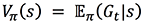Recall from previous section that Gt is defined by the cumulative sum of rewards starting from current time step t up to final time step T. Replacing Gt by the right side of the equation defined in the previous section and moving rt+1 out of the summation, you get: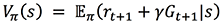Replacing the expectation formula by a sum over all probabilities yields: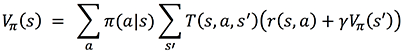The latter is called the Bellman equation; it enables you to compute V𝜋(s) for an arbitrary 𝜋 based on T and r, computing the new value of V at time step t based on the previous value of V at time step t+1, recursively. You can estimate T and r by counting all the occurrences of observed transitions and rewards in a set of observed interactions between the agent and the environment. T and r define the model of the MDP.

Now you know how to evaluate a policy, you can extend the notion of state value function to the notion of state-action value function Q(s,a) to define an optimal policy: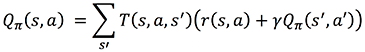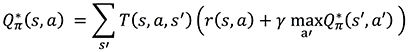The latter is called the Bellman Optimality equation; it estimates the value of taking a particular action a in a particular state s assuming you’ll take the best actions thereafter. If you transform the Bellman Optimality equation into an assignment function you get an iterative algorithm that, assuming you know T and r, is guaranteed to converge to the optimal policy with random sampling of all possible actions in all states. Because you can apply this equation to states in any order, this iterative algorithm is referred to as asynchronous dynamic programming.

For systems in which you can easily compute or sample T and r, this approach is sufficient and is referred to as model-based RL because you know (previously computed) the transition dynamics (as characterized by T and r). But for most real-case problems, it is more convenient to produce stochastic estimates of the Q-values based on experience accumulated so far, so the RL agent learns in real time at every step and ultimately converges toward true estimates of the Q-values. To this end, combine asynchronous dynamic programming with the concept of moving average: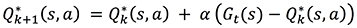and replace Gt(s) by a sampled value as defined in the Bellman Optimality equation: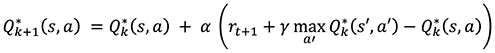The latter is called temporal difference learning; it enables you to update the moving average Q*k+1(s,a) based on the difference between Q*k(s,a) and Q*k(s’,a’). This post shows a one-step temporal difference, as used in the most straightforward version of the Q-learning algorithm, but you could extend this equation to include more than one step (n-step temporal difference). Again, some theorems exist that prove Q-learning converges to the optimal policy 𝜋* assuming infinite random action selection.

### RL action-selection strategy

You can alleviate the infinite random action selection condition by using a more efficient random action selection strategy such as ε-Greedy to increase sampling of states frequently encountered in good policies and decrease sampling of less valuable states. In ε-Greedy, the agent selects a random action with probability ε, and the rest of the time (that is, with probability 1 – ε), the agent selects the best action according to the latest Q-values, which is defined as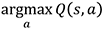.

This strategy enables you to balance exploiting the latest Q-values with exploring new states and actions. Generally, ε is chosen to be large at the beginning to favor exploration of state-action space, and progressively reduced to a smaller value. For example, ε = 1% to exploit Q-values most of the time yet keep exploring and potentially discovering better policies.

### Combining deep learning and reinforcement learning

In many cases, for example when playing chess or Go, the number of states, actions, and combinations thereof is so large that the memory and time needed to store the array of Q-values is enormous. There are more combinations of states and actions in the game Go than known stars in the universe. Instead of storing Q-values for all states and actions in an array which is impossible for Go and many other cases, deep RL attempts to generalize experience from a subset of states and actions to new states and actions. The following diagram is a comparison of Q-learning with look-up tables vs. function approximation.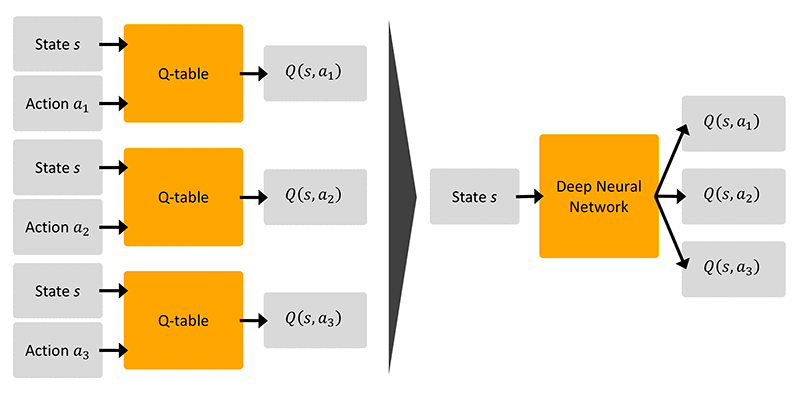Deep Q-learning uses a supervised learning approximation to Q(s,a) by using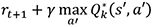as the label, because the Q-learning assignment function is equivalent to a gradient update of the general form x  = x – ∝∇J (for any arbitrary x) where: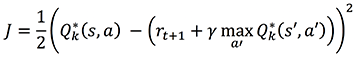The latter is called the Square Bellman Loss; it allows you to estimate Q-values based on generalization from mapping states and actions to Q-values using a deep learning network, hence the name deep Q-learning.

Deep Q-learning is not guaranteed to converge anymore because the label used for supervised learning depends on current values of the network weights, which are themselves updated based on learning from the labels, hence a problem of broken ergodicity. But this approach is often good enough in practice and can generalize to the most complex RL problems (such as autonomous driving, robotics, or playing Go).

In deep RL, the training set changes at every step, so you need to define a buffer to enable batch training, and schedule regular refreshes as experience accumulates for the agent to learn in real time. This is called experience replay and can be compared to the process of learning while dreaming in biological systems: experience replay allows you to keep optimizing policies over a subset of experiences (st, at, rt+1, st+1)n kept in memory.

This closes our theoretical journey on reinforcement learning and in particular the popular Deep Q-learning method, which implied several flavors of statistical learning, namely asynchronous dynamic programming, moving average, ε-Greedy and deep learning. Several RL variants exist in addition to the standard Deep Q-learning method, and Amazon SageMaker RL provides most of them out of the box, so after understanding one you will be able to test most of them using Amazon SageMaker RL.

## Implementing a custom RL model in Amazon SageMaker

Application of reinforcement learning in financial services has received attention because better financial decisions can lead to high dollar return. In this post I implement a financial trading bot to demonstrate how to develop an agent that looks at the price of an asset over the past few days and decide whether to buy, sell, or do nothing, given the goal to maximize long-term profit.

I will benchmark this approach with another approach in which I combine reinforcement learning with recurrent deep neural networks for time series forecasting. In this second approach, the agent uses insights from multi-step horizon forecasts to make trading decisions and learn policies based on forecasted sequences of events rather than past sequences of events.

The data is a randomized version of a publicly available dataset on Yahoo Finance and represents price series for an arbitrary asset. For example, you can think of this data as the price of an EC2 Spot Instance or the market value of a publicly traded stock.

### Setting up a built-in preset for deep Q-learning

In Amazon SageMaker RL, a preset file configures the RL training jobs and defines the hyperparameters for the RL algorithms. The following preset, for example, implements and parameterizes a deep Q-learning agent, as described in the previous section. It sets the number of steps to 2M. For more information about Amazon SageMaker RL hyperparameters and training jobs configuration, see Reinforcement Learning with Amazon SageMaker RL.

``````# Preset file in Amazon SageMaker RL

#################
# Graph Scheduling
#################

schedule_params = ScheduleParameters()
schedule_params.improve_steps = TrainingSteps(2000000)
schedule_params.steps_between_evaluation_periods = EnvironmentSteps(10000)
schedule_params.evaluation_steps = EnvironmentEpisodes(5)
schedule_params.heatup_steps = EnvironmentSteps(0)

############
# DQN Agent
############

agent_params = DQNAgentParameters()

# DQN params
agent_params.algorithm.num_steps_between_copying_online_weights_to_target = EnvironmentSteps(100)
agent_params.algorithm.discount = 0.99
agent_params.algorithm.num_consecutive_playing_steps = EnvironmentSteps(1)

# NN configuration
agent_params.network_wrappers['main'].learning_rate = 0.00025
agent_params.network_wrappers['main'].replace_mse_with_huber_loss = False

# ER size
agent_params.memory.max_size = (MemoryGranularity.Transitions, 40000)

# E-Greedy schedule
agent_params.exploration.epsilon_schedule = LinearSchedule(1.0, 0.01, 200000)

#############
# Environment
#############

##################
# Manage resources
##################

preset_validation_params = PresetValidationParameters()
preset_validation_params.test = True

graph_manager = BasicRLGraphManager(agent_params=agent_params, env_params=env_params,
schedule_params=schedule_params, vis_params=VisualizationParameters(),
preset_validation_params=preset_validation_params)``````

### Setting up a custom OpenAI Gym RL environment

In Amazon SageMaker RL, most of the components of an RL Markov Decision Process as described in the previous section are defined in an environment file. You can connect open-source and custom environments developed using OpenAI Gym, which is a popular set of interfaces to help define RL environments and is fully integrated into Amazon SageMaker. The RL environment can be the real world in which the RL agent interacts or a simulation of the real world. You can simulate the real world by a daily series of past prices. To formulate the bidding problem into an RL problem, you need to define each of the following components of the MDP:

• Environment – Custom environment that generates a simulated price with daily and weekly variations and occasional spikes
• State – Daily price of the asset over the past 10 days
• Action – Sell or buy the asset, or do nothing, on a daily basis
• Goal – Maximize accumulated profit
• Reward – Positive reward equivalent to daily profit, if any, and a penalty equivalent to the price paid for any asset purchased

A custom file called `TradingEnv.py` specifies all these components. It is located in the `src/` folder and called by the preset file defined earlier. In particular, the size of the state space (n = 10 days) and the action space (m = 3) are defined using OpenAI Gym through the following lines of code:

``````# Custom environment file in Open AI Gym and Amazon SageMaker RL

def __init__(self,casset = 'asset_prices', span = 10 ):

self.asset = asset
self.span = span

datafile = DATA_DIR + asset + '.csv'
self.observations = self.getObservations(datafile)

# Number of time steps in data file
self.steps = len(self.observations) - 1

# Output filename
self.csv_file = CSV_DIR

# Action
self.action_space = gym.spaces.Discrete(3)

# Observation space is defined from the data min and max
self.observation_space = gym.spaces.Box(low = -np.inf, high = np.inf, shape = (self.span,), dtype = np.float32)

(…)
``````

The goal is translated into a quantitative reward function, as explained in the previous section, and this reward function is also formulated in the environment file. See the following code:

``````# Definition of reward function
def step(self, action):

if action == 1:
self.inventory.append(self.observations[self.t])
margin = 0
reward = 0
# SELL:
elif action == 2 and len(self.inventory) > 0:
bought_price = self.inventory.pop(0)
margin = self.observations[self.t] - bought_price
reward = max(margin, 0)
# SIT:
else:
margin = 0
reward = 0

self.total_profit += margin

# Increment time
self.t += 1

# Store state for next day
obs = self.getState(self.observations, self.t, self.span + 1, self.mode)

# Stop episode when reaches last time step in file
if self.t >= self.steps:
done = True
else:
done = False

(…)

return obs, reward, done, info
``````

### Launching an Amazon SageMaker RL estimator

After defining a preset and RL environment in Amazon SageMaker RL, you can train the agent by creating an estimator following a similar approach to when using any other ML estimators in Amazon SageMaker. The following code shows how to define an estimator that calls the preset (which itself calls the custom environment):

``````# Definition of the RL estimator in Amazon SageMaker RL

estimator = RLEstimator(source_dir='src',
entry_point=entryfile,
dependencies=["common/sagemaker_rl"],
toolkit=RLToolkit.COACH,
toolkit_version='0.11.0',
framework=RLFramework.MXNET,
role=role,
train_instance_count=1,
train_instance_type='ml.c5.9xlarge',
output_path=s3_output_path,
base_job_name=job_name_prefix,
``````

You would generally use a `config.py` file in Amazon SageMaker RL to define global variables, such as the location of a custom data file in the Amazon SageMaker container. See the following code:

``````# Configuration file (config.py) used to define global variables in Amazon SageMaker RL

import os
CUR_DIR = os.path.dirname(__file__)
DATA_DIR = CUR_DIR + '/datasets/'
CSV_DIR = '/opt/ml/output/data/simulates.csv'``````

## Training and evaluating the RL agent’s performance

This section demonstrates how to train the RL bidding agent in a simulation environment constructed using seven years of daily historical transactions. You can evaluate learning during the training phase and evaluate the performance of the trained RL agent at generalizing on a test simulation, here constructed using three additional years of daily transactions. You can assess  the agent’s ability to learn and generalize by visualizing the accumulated reward and accumulated profit per episode during the training and test phases.

### Evaluating learning during training of the RL agent

In reinforcement learning, you can train an agent by simulating interactions (st, at, rt+1, st+1) with the environment and computing the cumulative return Gt, as described previously. After an initial exploration phase, as the value of ε in ε-Greedy progressively decreases to favor exploitation of learned policies, the total accumulated reward in a given episode accounts for how good the policy followed was. Thus, the total accumulated reward compared between episodes enables you to evaluate the relative quality of the policies learned. The following graphs show the reward and profit accumulated by the RL agent during training simulations. The x-axis is the episode index and the y-axis is the reward or profit accumulated per episode by the RL agent.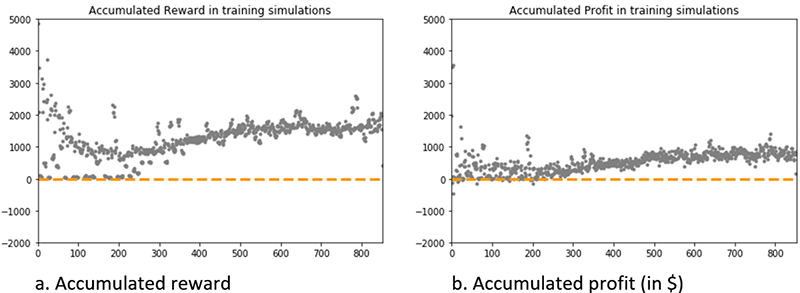The graph on accumulated reward shows a trend with essentially three phases: relatively large fluctuations during the first 300 episodes (exploration phase), steady growth between 300 and 500 episodes, and a plateau (500–800 episodes) in which the total accumulated reward has reached convergence and revolves around a relatively stable value of \$1,800.

Consistently, the accumulated profit is approximately zero during the first 300 episodes and revolves around a relatively stable value of \$800 after episode 500.

Together, these results indicate that the RL agent has learned to trade the asset profitably, and has converged toward a stable behavior with reproducible policies to generate profit over the training dataset.

### Evaluating generalization on a test set of the RL agent

To evaluate the agent’s ability to generalize to new interactions with the environment, you can constrain the agent to exploit the learned policies by setting the value of ε to zero and simulate new interactions (st, at, rt+1, st+1) with the environment.

The following graph shows the profit generated by the RL agent during test simulations. The x-axis is the episode index and the y-axis is profit accumulated per episode by the RL agent.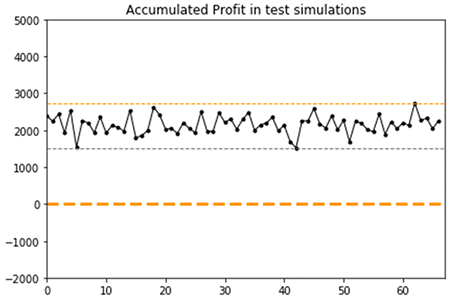The graph shows that after three years of new daily prices for the same asset, the trained agent tends to generate profits with a mean of \$2,200 and standard deviation of \$600 across 65 test episodes. In contrast to the training simulations, you can observe a steady mean across all 65 test episodes simulated, indicating a strong convergence of the policies learned.

The graph confirms that the RL agent has converged and learned reproducible policies to trade the asset profitably and can generalize to new prices and trends.

## Benchmarking a deep recurrent RL agent

Finally, I benchmarked the results from the previous deep RL approach with a deep recurrent RL approach. Instead of defining a state as a window of the past 10 days, an RNN for time series forecasting encodes the state as a 10-day horizon forecast, so the agent benefits from an advisor on future expected prices when learning optimal trading policies for maximizing long-term return.

### Implementing a forecast-based RL state space

The following code implements a simple RNN for time series forecasting, following the post Forecasting financial time series with dynamic deep learning on AWS:

``````# Custom function to define a RNN for time series forecasting

def trainRNN(self, data, lag, horiz, nfeatures):

# Set hyperparameters
nunits = 64                         # Number of GRUs in recurrent layer
nepochs = 1000                 # Number of epochs
d = 0.2                                 # Percent of neurons to drop at each epoch
optimizer = 'adam'            # Optimization algorithm
activ = 'elu'                         # Activation function of neurons
activr = 'hard_sigmoid'.   # Activation function of recurrent layer
activd = 'linear'                  # Dense layer's activation function
lossmetric = 'mean_absolute_error'  # Loss function for gradient descent
verbose = False                  # Print results on standard output channel

# Prepare data
df = data
df = df.diff()

data = df.as_matrix()
lags = []
horizons = []
nsample = len(data) - lag - horiz
for i in range(nsample):
lags.append(data[i: i + lag , -nfeatures:])
horizons.append(data[i + lag : i + lag + horiz, -1])
lags = np.array(lags)
horizons = np.array(horizons)
lags = np.reshape(lags, (lags.shape, lags.shape, nfeatures))

# Design RNN architecture
rnn_in = Input(shape = (lag, nfeatures), dtype = 'float32', name = 'rnn_in')
rnn_gru = GRU(units = nunits, return_sequences = False, activation = activ, recurrent_activation = activr, dropout = d)(rnn_in)
rnn_out = Dense(horiz, activation = activd, name = 'rnn_out')(rnn_gru)
model = Model(inputs = [rnn_in], outputs = [rnn_out])
model.compile(optimizer = optimizer, loss = lossmetric)

# Train model
fcst = model.fit({'rnn_in': lags},{'rnn_out': horizons}, epochs=nepochs,verbose=verbose)
``````

And the following function redirects the agent to an observation based on lag or forecasted horizon (the two types of RL approaches discussed in this post), depending on the value of a custom global variable called mode:

``````# Custom function to define a state at time t

def getState(self, observations, t, lag, mode):

# Mode "past": State at time t defined as n-day lag in the past
if mode == 'past':
d = t - lag + 1
block = observations[d:t + 1] if d >= 0 else -d * [observations] + observations[0:t + 1] # pad with t0
res = []
for i in range(lag - 1):
res.append(self.sigmoid(block[i + 1] - block[i]))
return np.array([res])

# Mode "future": State at time t defined as the predicted n-day horizon in the future using RNN
elif mode == 'future':
d = t - lag + 1
block = observations[d:t + 1] if d >= 0 else -d * [observations] + observations[0:t + 1] # pad with t0
horiz = rnn.predict(block)
res = []
for i in range(lag - 1):
res.append(self.sigmoid(horiz[i + 1] - horiz[i]))

``````

### Evaluating learning during training of the RNN-based RL agent

As in the previous section for the RL agent in which a state is defined as the lag of the past 10 observations (referred to as lag-based RL), you can evaluate the relative quality of the policies learned by the RNN-based agent by comparing the total accumulated reward between episodes.

The following graphs show the reward and profit accumulated by the RNN-based RL agent during training simulations. The x-axis is the episode index and the y-axis is the reward or profit accumulated per episode by the RL agent.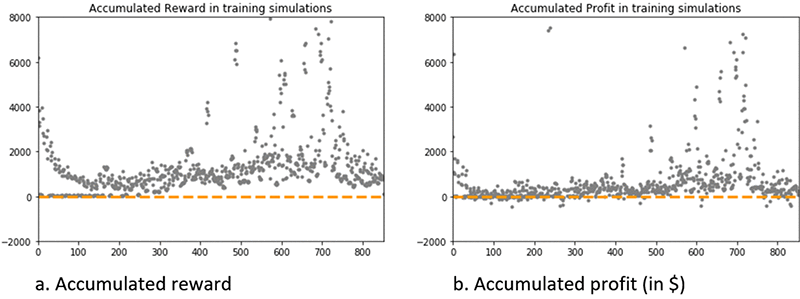The graph on accumulated reward shows a steady growth after episode 300, and most episodes after episode 500 result in an accumulated reward of approximately \$1,000. But several episodes result in a much higher reward of up to \$8,000.

The accumulated dollar profit is approximately zero during the first 300 episodes, revolves around a positive value after episode 500, with again several episodes where the generated profit reaches up to \$7,500.

Together, these results indicate that the RL agent has learned to trade the asset profitably, and has converged toward a behavior with policies that generate profit either around \$500–800, or some much higher values up to \$7,500, over the training dataset.

### Evaluating generalization on a test set of the RNN-based RL agent

The following graph shows the profit in dollars generated by the RNN-based RL agent during test simulations. The x-axis is the episode index and the y-axis is the profit accumulated per episode by the RL agent.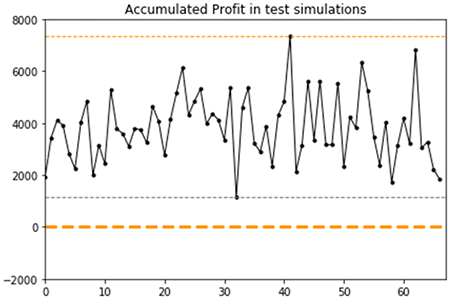The graph shows that the RNN-based RL agent can generalize to new prices and trends. Consistent with the observations made during training, it shows that after three years of new daily prices for the same asset, the trained agent tends to generate profits around \$2,000, or some much higher profit up to \$7,500. This dual behavior is characterized by an overall mean of \$4,900 and standard deviation of \$3,000 across the 65 test episodes.

The minimum profit across all 65 test episodes simulated is \$1,300, while it was \$1,500 for the lag-based RL approach. The maximum profit is \$7,500, with frequent accumulated profit over \$5,000, while it was only \$2,800 for the lag-based RL approach. Thus, the RNN-based RL approach results in higher volatility compared to the lag-based RL approach, but exclusively toward higher profits which is a better outcome given the goal of the RL problem is to generate profit.

The results between the lag-based RL agent and the RNN-based RL agent confirm that the RNN-based RL agent is more beneficial. It has learned reproducible policies to trade the asset that are either similar to, or significantly outperform, the policies learned by the lag-based agent for generating profit.

## Conclusion

In this post, I introduced the motivations and underlying theoretical concepts behind deep reinforcement learning, an ML solution framework that enables you to learn and apply optimal policies given a long-term objective. You have seen how to use Amazon SageMaker RL to develop and train a custom deep RL agent to make smart decisions in real time in an arbitrary bidding environment.

You have also seen how to evaluate the performance of the deep RL agent during training and test simulations, and how to benchmark its performance against a more advanced, deep recurrent RL based on RNN for time series forecasting.

This post is for educational purposes only. Past trading performance does not guarantee future performance. The loss in trading can be substantial; investors should use all trading strategies at their own risk.

For more information about popular RL algorithms, sample codes, and documentation, you can visit Reinforcement Learning with Amazon SageMaker RL.Jeremy David Curuksu is a data scientist at AWS and the global lead for financial services at Amazon Machine Learning Solutions Lab. He holds a MSc and a PhD in applied mathematics, and was a research scientist at EPFL (Switzerland) and MIT (US). He is the author of multiple scientific peer-reviewed articles and the book Data Driven, which introduces management consulting in the new age of data science.

# Plug yourself into AI and don't miss a beat

Toronto AI is a social and collaborative hub to unite AI innovators of Toronto and surrounding areas. We explore AI technologies in digital art and music, healthcare, marketing, fintech, vr, robotics and more. Toronto AI was founded by Dave MacDonald and Patrick O'Mara.